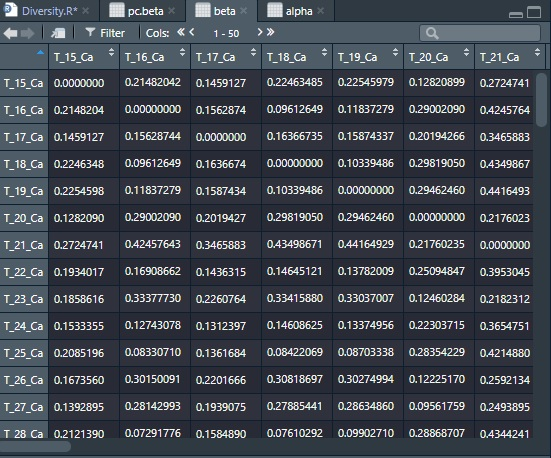# Interpretation of the PERMANOVA results

Hi,

I have followed the "Moving pictures" Tutorial to generate the PERMANOVA results using the `beta-group-significance` command.

And I am confused about the PERMANOVA results:

1. For example, for "Distances to gut", what does the boxplot of gut samples mean? It's the distance of what? gut sample to gut sample?
2. And also, what does this n mean? The total sample size is 31, but some of n here is larger than 31.
3. And what is the sample size in the pairwise permanova results?

Thanks!

The results can be found here.

Welcome to the forum, @spencer886!
Unlike alpha diversity metrics, which estimate diversity within a certain niche /sample, beta diversity is shaped to compare different nuches/samples. So, basically you have a matrix with distances of each sample in you feature table to all other samples.
On the graph, you can see distances of gut samples to gut samples (all distances between samples in gut group), as well as distances between samples from different groups (rest of the boxes).
Since each sample from one group compared to each sample within other (the same as well) group, number of distances is higher than number of samples.
See this post for better explanation
When comparing group A with group B, sampling size will be number of samples in your metadata that belongs to compared groups.

Attached random photo from the internet as an example1 Like

Thanks, Timur! This is really helpful! Now I understand the plot.

So we have 31 samples, 8 from gut, 8 from left palm, 6 from right palm, and 9 from tongue.
n = 28 is the number of distances between the gut samples, it's n = 87/2.
n = 64 is the number of distances between gut samples and left palm samples for this plot: n = 8
8.

And the sample size in the pairwise permanova results is just the sum of sample size from two different groups, i.e., group 1: gut, group 2: left palm, we have sample size = 8 + 8 = 16.

1 Like

This topic was automatically closed 31 days after the last reply. New replies are no longer allowed.